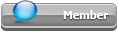## Featured Articles

Check out the latest featured articles.## File Library0# Constant Rate Drying Equation During Flash/pneumatic Drying

constant rate drying flash drying pneumatic drying

|

### #1cheminst

cheminst

Veteran Member

•• Members
•• 33 posts

Posted 20 June 2019 - 12:33 AM

I had this problem while calculating the constant drying rate during flash/ pneumatic drying.

Drying rate can be calculated by two ways. These are the equations I found in literature for calculating the constant drying rate for flash/pneumatic drying. (Free moisture is only evaporated. particle temperature normally doesn't exceed 50 Celsius)

w = drying rate (kg/ m2 s)

k = mass transfer coefficient

Ts = Solid temperature = 303 K = 30 C

Tg = Gas temperature = 413 K = 140 C

Mw = molecular weight of H2O (18 e-3 kg/mol)

Mg = molecular weight of dry gas (30 e-3 kg/mol)

R = Gas constant (8.314 J/mol K)

P = atmospheric pressure (101325 Pa)

Psat = Saturation water vapor pressure at solid temperature, 303 K = 4247 Pa

Drying gas has 12% moisture by volume.

PH2O = partial pressure of gas =101325*0.12 = 12159 Pa

H = humidity in gas (H2O kg/ dry gas kg) = (0.12*18) / (0.88*30) = 0.0818

1) First Method (for single particle)

w = k (Psat *Mw / R*Ts  -   PH2O*Mw / R*Tg)

However, the drying rate is negative. since (4247/303 - 12159/413) < 0

2) Second method (for bulk of the particles)

w = k { [Mw*Psat /  Mg*(P - Psat) ] -   H }

However drying rate is negative. since  [ 18*4247/30*(101325 - 4247) ] - 0.0818  < 0 is negative.

Can anyone please explain why the drying rate is negative. Is there anything wrong with the equations or my calculations?

### #2PhilippM

PhilippM

Gold Member

•• Members
•• 98 posts

Posted 31 July 2019 - 03:27 PM

You wrote that you found these equations in literature. Can you provide a source citation? That might make it easier to understand the problem.

### Similar Topics